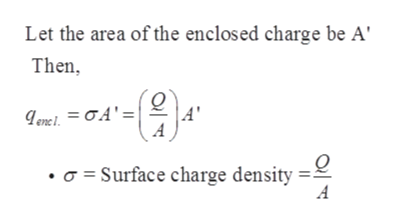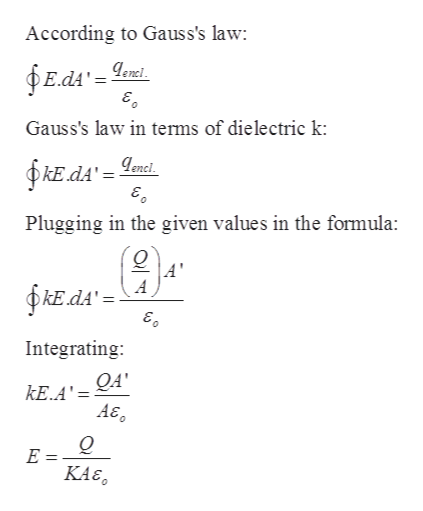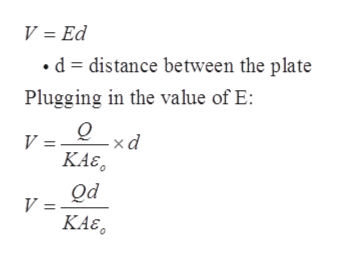# Part A throught C pleaseA parallel-plate capacitor has the volume between its plates filled with plastic with dielectric constant K . The magnitude of the charge on each plate is Q . Each plate has area A , and the distance between the plates is d Part A Use Gauss's law to calculate the magnitude of the electric field in the dielectric. Express your answer in terms of the given quantities and appropriate constants. Part B Use the electric field determined in part A to calculate the potential difference between the two plates. Part C Use the result of part B to determine the capacitance of the capacitor. Express your answer in terms of the given quantities and appropriate constants.

Question
8 views

A parallel-plate capacitor has the volume between its plates filled with plastic with dielectric constant K . The magnitude of the charge on each plate is Q . Each plate has area A , and the distance between the plates is d

Part A Use Gauss's law to calculate the magnitude of the electric field in the dielectric. Express your answer in terms of the given quantities and appropriate constants.

Part B Use the electric field determined in part A to calculate the potential difference between the two plates.

Part C Use the result of part B to determine the capacitance of the capacitor. Express your answer in terms of the given quantities and appropriate constants.

check_circle

Step 1

Given:

Magnitude of charge on each plate = Q

Area of each plate = A

Dielectric constant = K

Distance between the plate = dhelp_outlineImage TranscriptioncloseLet the area of the enclosed charge be A Then genel. = CA' = a Surface charge density A fullscreen
Step 2

(a)help_outlineImage TranscriptioncloseAccording to Gauss's law: Edd'= Leni Gauss's law in terms of dielectric k: PkE.ds'= Lnct Plugging in the given values in the formula: A' A E dA' Integrating: QA' kE..A'= A& Q E KAE fullscreen
Step 3

(b)

The relation between the potential d...help_outlineImage TranscriptioncloseV = Ed «d = distance between the plate Plugging in the value of E: V =__xd КАt, Od V KAE fullscreen

### Want to see the full answer?

See Solution

#### Want to see this answer and more?

Solutions are written by subject experts who are available 24/7. Questions are typically answered within 1 hour.*

See Solution
*Response times may vary by subject and question.
Tagged in

### Electric Charges and Fields